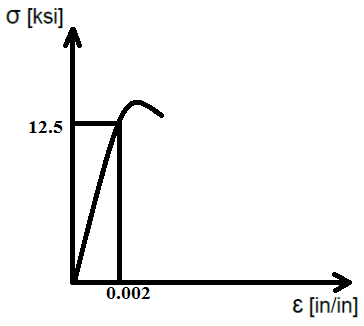# The elastic portion of the tension stress-strain diagram for an aluminium alloy is shown in the...

## Question:

The elastic portion of the tension stress-strain diagram for an aluminium alloy is shown in the figure. The specimen used for the test has a gauge length of 2 in. If the applied load is 10 kip, determine the new diameter of the specimen. The shear modulus is G=3800 ksi.## Hooke's Law:

Hooke's law states that when the stress-strain graph is plotted, the stress is directly proportional to the strain upto the limit of proportionality. The proportionality constant is know as Young's modulus of elasticity. The slope of the curve gives us the modulus of elasticity of the material.

{eq}E = \dfrac{\sigma}{\varepsilon} {/eq}

Where,

• {eq}\sigma {/eq} is the stress.
• {eq}\varepsilon {/eq} is the strain.
• E is the young's modulus.

We're given the following information in the problem:

The gauge length is, {eq}L_o = 2\ in {/eq}

The load applied is, {eq}P = 10\ kip {/eq}

Let...

Become a Study.com member to unlock this answer! Create your account